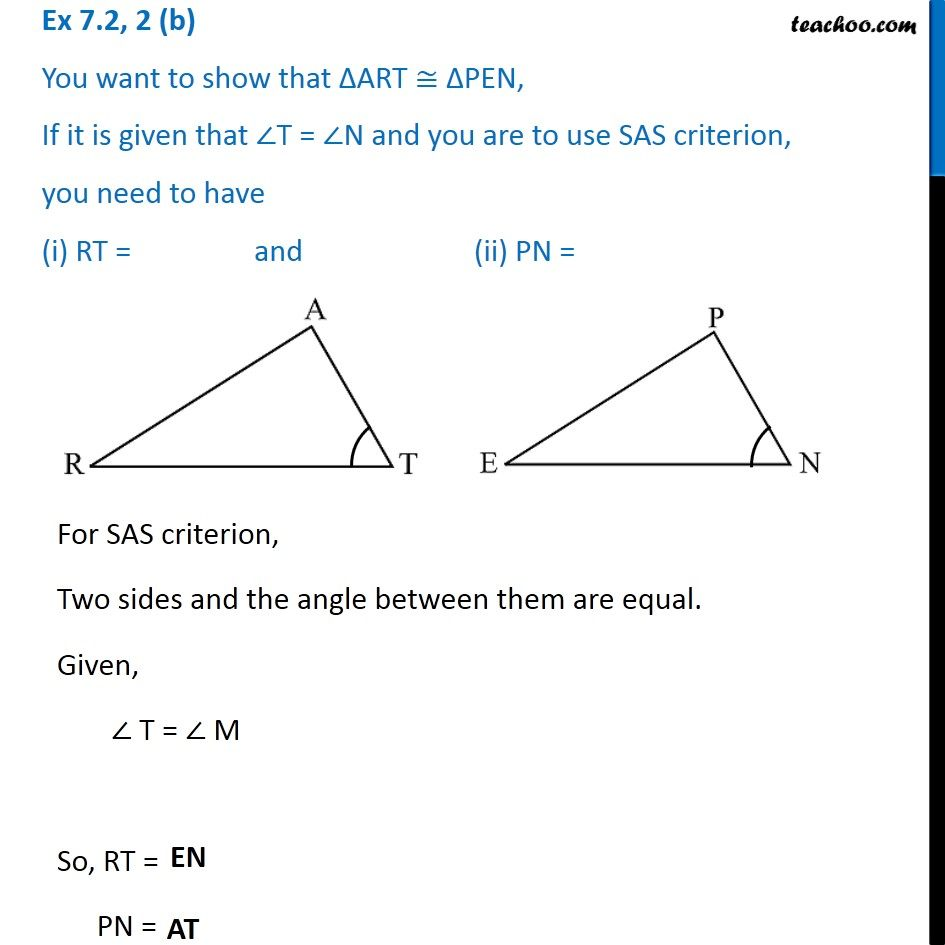1. Chapter 7 Class 7 Congruence of Triangles
2. Serial order wise
3. Ex 7.2

Transcript

Ex 7.2, 2 (b) You want to show that ∆ART ≅ ∆PEN, If it is given that ∠T = ∠N and you are to use SAS criterion, you need to have (i) RT = and (ii) PN =For SAS criterion, Two sides and the angle between them are equal. Given, ∠ T = ∠ M So, RT = PN =